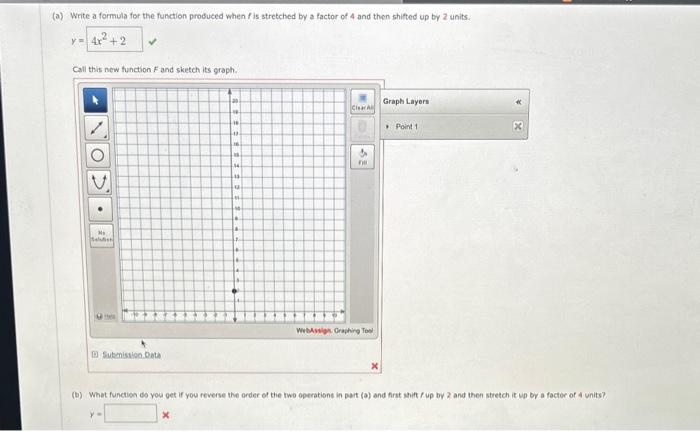Home / Expert Answers / Algebra / a-write-a-formula-for-the-function-produced-when-f-is-stretched-by-a-factor-of-4-and-then-shifted-pa343

# (Solved): (a) Write a formula for the function produced when f is stretched by a factor of 4 and then shifted ...(a) Write a formula for the function produced when is stretched by a factor of 4 and then shifted up by 2 units. Call this new tunction and sketch its graph. (b) What funetion do you get if you reverse the order of the two operations in part (a) and fint sift up by 2 and then stretch it up by a factor of a units?

We have an Answer from Expert